Dr. J's Maths.com
Where the techniques of Maths
are explained in simple terms.

Applications of Calculus - Graphs of motion in one direction.
Test Yourself 1.

 The questions on this page focus on: 1. given a displacement graph. 2. given a velocity graph. 3. given an acceleration graph.

 Given displacement graph. 1.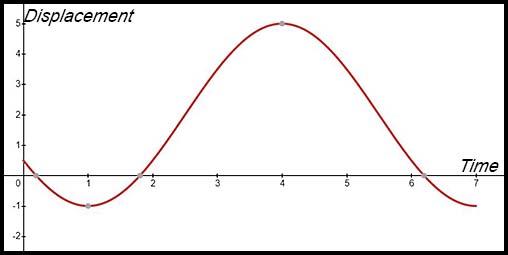A particle moves along a straight line for 7 seconds starting at a fixed point S at time t = 0. The graph of x(t) denoting the position of P at time t is shown above. (i) Where did the particle start from approximately? (ii) In which direction is the particle travelling initially? (iii) At approximately what times does the particle have zero velocity? (iv) At approximately what time is the distance from S the greatest? (v) Is the particle speeding up or slowing down when t = 5? Explain your answer. Answers.(i) Particle started at S = 0.5 (approx). (ii) In a negative direction as the gradient is negative. (iii) At t = 1 and t = 4.(iv) At t = 4 seconds.(v) At t = 5 the gradient is negative and the concavity is still negative. So the particle is speeding up in a negative direction - so slowing down in a positive direction. 2. The graph showing the motion of a particle over the first 3 seconds is shown below (displacement in metres).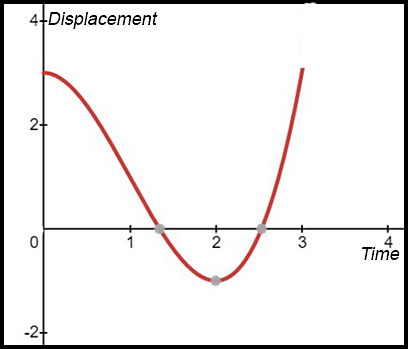(i) Describe the initial motion of the particle. (ii) Describe the motion of the particle at t = 0.5 secs. (iii) Describe the motion of the particle at t = 1.5 secs. (iv) Describe the motion of the particle at t = 2.5 secs. 3. 4. Sketch a graph of displacement against time given that, during the period of motion, the displacement is always negative, the velocity is always negative and the acceleration is always positive. 5. Sketch a graph of displacement against time given that, during the period of motion, the displacement is always positive, the velocity is always positive and the acceleration is always negative. Given velocity graph. 6. The diagram below describes the velocity of a particle (in m/sec) over the first 4 seconds.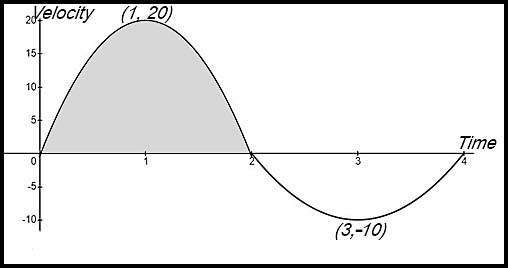(i) What is the velocity of the particle after 1 second? (ii) What is the acceleration of the particle after 3 seconds? (iii) When does the particle change direction? (iv) Use 4 applications of the Trapezoidal Rule to estimate the displacement of the particle after the first 4 seconds. Answers.(i) 20 m/sec.(ii) Acc = -10 m/sec2. (iii) At t = 2.(iv) Displacement = 10 m. 7.The graph above shows the velocity of a particle against time of travel. Initially the particle was at the origin. (i) When is the particle furthest from the origin? (ii) How far and in what direction is the particle from the origin after 7 seconds? (iii) Sketch the acceleration of the particle from t = 0 to t = 7 seconds. Answers.(i) Furthest at 4 secs.(ii) After 7 secs, 4 m to right of origin. 8. The graph shows the velocity v (in metres/sec) of a particle, moving from the origin, as a function of time t.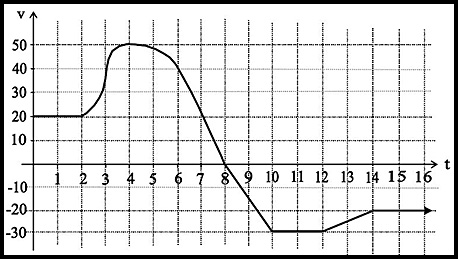(i) At approximately what time is the acceleration greatest? Justify your answer. (ii) Use the trapezoidal rule with 4 sub-intervals to calculate the total distance travelled by the particle between t = 0 and t = 8. (iii) Given that the particle is initially at the origin, calculate the time at which it will return to the origin. Give reasons. (iv) Describe the motion of the particle. Answers.(i) Max acceleration at t = 3.(ii) Distance = 240 m. (iii) Returns to origin at t = 18 secs. 9.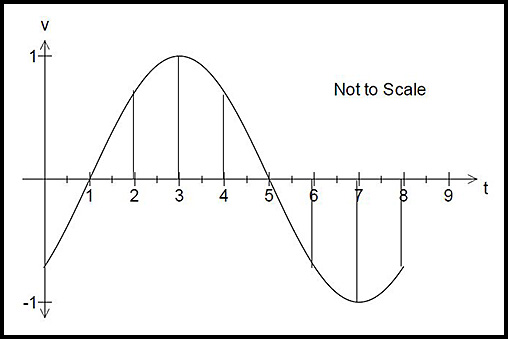The diagram above shows the velocity-time graph for a particle moving in a straight line. State the times between t = 0 and t = 8 at which: (i) the velocity is zero. (ii) the acceleration is zero. (iii) the speed is increasing. (This is hard - think about it). Answers.(i) v = 0 at t = 1 and 5 secs. (ii) t = 3 and 7. (iii) 1 < t < 3 (v & a both positive) and 5 < t < 7 (v & a both negative). 10. An object is moving along in a straight line. The graph below shows the velocity (in m/sec),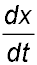, of the object as a function of time t. The coordinates of the points on the graph are A (1, 0), B (2, 8), C (3, 0), D (3.5, -3), E (4, 0) and F (5, 6). The velocity is constant for t ≥ 5.(i) The object is initially at the origin. During which time(s) is the displacement of the object decreasing? (ii) Draw a sketch of the graph representing the acceleration of the particle. (iii) Using the Trapezoidal Rule, show that the distance travelled between t = 1 and t = 4 is 9.5 m. (iv) Sketch the displacement, x, as a function of time. 11.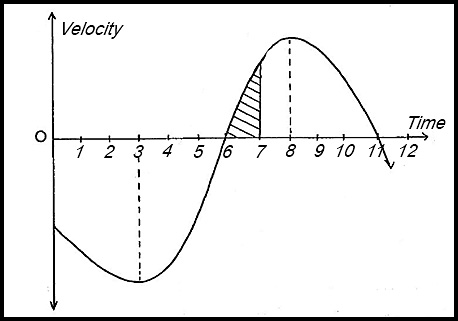The diagram above shows the velocity-time graph for a particle moving in a straight line. (i) When is the particle at rest? (ii) When is the acceleration of the particle zero? (iii) When does the maximum acceleration of the particle occur? (iv) What does the shaded region represent? (v) Is the particle further from its initial position at t = 6 or at t = 11? Give reasons for your answer. 12. Below is the graph of a particle’s velocity v in m/sec plotted against time t in seconds. The particle’s motion lasts 10 seconds.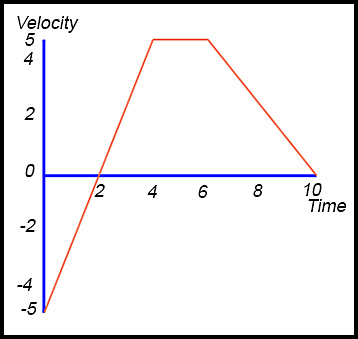(i) State the time(s) when the particle is at rest. (ii) Initially the particle is at the origin. Find the next time the particle is at the origin. (iii) Find the final displacement of the particle. (iv) Find the total distance travelled by the particle. Given acceleration graph. 13.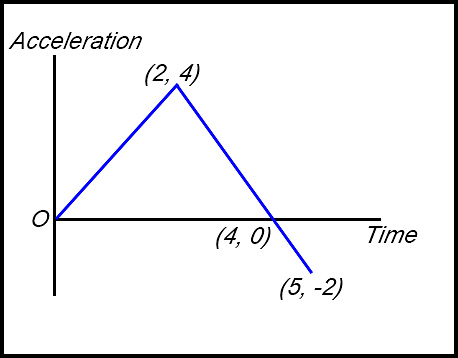The graph shows the acceleration of a particle as a function of time t for the period 0 ≤ t ≤ 5. (i) What is the maximum acceleration of the particle in this period? (ii) At what time in this period does the velocity reach its maximum? State what that maximum acceleration is. 14.A particle moves along the x-axis. Initially it is at rest at the origin. The graph shows the acceleration a of the particle as a function of time 0 ≤ t ≤ 5. (i) Write down the time at which the velocity of the particle is a maximum. (ii) What is the final velocity of the particle at t = 5? (ii) At what time during the interval 0 ≤ t ≤ 5 is the particle furthest from the origin? Give brief reasons for your answer. Answer.(i) Max velocity at t = 2. (ii) Final velocity is -3 m/sec.(iii) Furthest from origin at t = 4. 15.